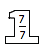### Home > MC1 > Chapter 9 > Lesson 9.3.1 > Problem9-126

9-126.

Multiple Choice: Which fraction below is equivalent to $\frac { 7 } { 8 }$? Explain how you can tell.

1. $\frac { 9 } { 10 }$

1. $\frac { 14 } { 8 }$

1. $\frac { 49 } { 56 }$

1. $\frac { 49 } { 64 }$

Which one of these is the original fraction multiplied by a Giant One?

The Giant One used is the one on the right.